# K-means clustering in python

## INTRODUCTION

k-means clustering is a method of vector quantization . It is a type of unsupervised learning , which is used when you have unlabeled data . The goal of this algorithm is to find groups in the data, with the number of groups represented by the variable K i.e., k-means clustering aims to partition n observations into k clusters in which each observation belongs to the  cluster with the nearest  mean .  The algorithm works iteratively to assign each data point to one of K groups based on the features that are provided . The results of theK-means clustering algorithm are as follows:

• The centroids of the K clusters that can be used to label new data
• Labels for the training data i.e., every data point gets assigned to a single cluster

## ALGORITHM

The algorithm works as follows:

• First we initialize k points, called means, randomly.
• We categorize each item to its closest mean and we update the mean’s coordinates, which are the averages of the items categorized in that mean so far.
• We repeat the process for a given number of iterations and at the end, we have our clusters.

### PSEUDO-CODE

The above algorithm in pseudocode:

```Initialize k means with random values

For a given number of iterations:
Iterate through items:
Find the mean closest to the item
Assign item to mean
Update mean

```

### MAIN CODE

Now,lets have a look at the code:-

1.First import the required libraries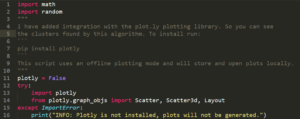2. create a point class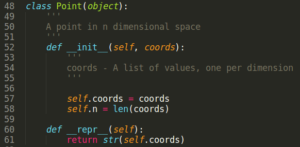3.now, write functions for generating random points and getting distance between points as follows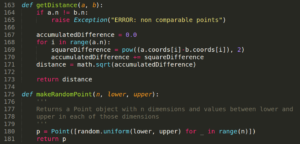4.Now,create a class cluster which clusters(groups) the points . it has the functions to calculate the centroid for a cluster and for updating the points in a cluster(in case , if there is a nearer centroid than the current centroid)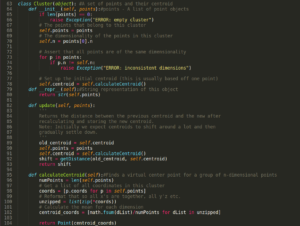5.write the k-means function which keeps updating the functions iteratively till a certain cutoff is reached .6.now, write a function to plot clusters(look at  my github for this function) . For 2 and  3 dimensions the output can be visualized and can be seen on the web browser . For higher dimensions(i.e.,for dimensions>3) plots cannot be plotted but the clusters can be seen in the output

7.finally , write a main function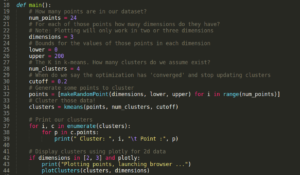8.The output is seen as shown below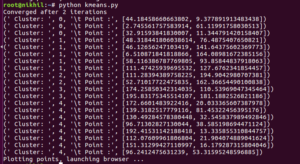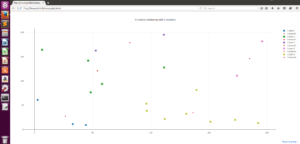For three dimensional points also we get 3d graphs but for dimensions above 3 the output can be seen only on terminal  .

It is one of the most important algorithm in machine learning

To get the whole code , please visit my github profile

Thank you

“The beautiful thing about learning is nobody can take it away from you”

### 1 Comment

#### DeepFace and Facenet for face recognition - projectsflix · July 1, 2019 at 5:35 pm

[…] check our article on k-means clustering here. […]

This site uses Akismet to reduce spam. Learn how your comment data is processed.